Published: 31 December 2016

# Vibration and instability analysis of fluid-conveying nanotubes embedded in visco-elastic medium with consideration of surface effect

Ya-Xin Zhen1
1School of Mathematics and Physics, North China Electric Power University, Beijing, 102206, China
Views 115

#### Abstract

We establish an analytical model to investigate the surface effects on the vibration and instability of fluid-conveying nanotubes embedded in visco-elastic medium. Based on nonlocal elastic theory and Euler-Bernoulli beam theory, the vibration equation of fluid-conveying nanotubes is established with considering three typical boundary conditions. The effects of both inner and outer surface layers on the nanotubes are taken into consideration and the Kelvin-Voigt model is introduced. The results show that the boundary conditions of system, the damping and elastic coefficient of the surrounding medium, thickness of nanotubes and aspect ratios have significant effects on the dynamic behaviors of the nanotubes. The damping parameter of the visco-elastic foundation causes an obvious reduction of the critical flow velocity. For smaller tube thickness, larger aspect ratio or higher elastic parameter of surrounding foundation, the stability of the nanotubes may be greatly enhanced. This article might be helpful for the design and improvement of nanotubes for fluid-conveying applications embedded in elastic medium in nanoelectromechanical systems and microelectromechanical systems.

## 1. Introduction

For the wide applications of nanotubes/nanopipes in nanoelectromechanical systems (NEMS) and microelectromechanical systems (MEMS), many attempts have been made to study their properties [1, 2]. Due to the hollow cylindrical geometry structure of nanotubes, they are expected to be used as nanofluidic devices and systems, with potential application in fluid filtration devices, biomimetic selective transport of ions, targeted drug delivery devices and so on [3-5].

As a small scale fluid-structure interaction system, the fluid-conveying nanotubes show higher sensitivity to the vibration characteristics. The research of flow-induced vibration and instability is of fundamental significance. In the past two decades, the literature on this topic has been constantly expanding. Many researchers have done much work in this area [6-12]. The systems they studied range from straight to curved configurations of nanotubes with either single wall or multi walled, linear and nonlinear vibration [13-16].

In classical continuum mechanics, the surface energy is usually small and negligible. But when materials and structures shrink to nanometers, it plays a critical role in their static or dynamical behavior due to the high specific surface area of nanomaterials . He and Lilley  studied the effects of surface tension on the static bending of nanowires. Farshi et al.  researched the vibration of nanotubes with consideration of surface effects utilizing Timoshenko beam model. Gheshlaghi and Hasheminejad  investigated the surface effects on the nonlinear vibration of nanobeams based on the Euler-Bernoulli beam theory including the von Kármán geometric nonlinearity.

The vibration and buckling instability of fluid-conveying nanotubes by accounting for surface layers were analyzed by Wang , who found that the natural frequencies and critical flow velocity are strongly related to the surface effect. With the increasing ratio of surface layer to volume (nanotube wall) at the nanoscale, the vibration characteristics of nanotubes conveying fluid were shown to exhibit distinct size dependences. Furthermore, the surface effect on the buckling and post-buckling behavior of a nanobeam with supported ends containing flowing fluid is investigated using a nonliner model .

In this paper, an analytical model is established to invesitigate the surface effect on the vibration and instability of fluid-conveying nanotubes embedded in viso-elastic medium by nonlocal Euler-bernoulli beam model. It will be shown that the current model will be helpful for designing the nanotubes for fluid-conveying applications embedded in visco-elastic medium in NEMS and MEMS.

## 2. Fundamental equations

The circular nanotube embedded in visco-elastic medium is modeled as a straight and slender nanobeam with length $L$, as shown in Fig. 1. From reference  we get the vibration equation of fluid-conveying nanotube embedded in visco-elastic medium based on nonlocal Euler-Bernoulli beam theory as follow:

1
$EI\frac{{\partial }^{4}w}{\partial {x}^{4}}+m\frac{{\partial }^{2}w}{\partial {t}^{2}}+{m}_{f}\left({u}^{2}\frac{{\partial }^{2}w}{\partial {x}^{2}}+2u\frac{{\partial }^{2}w}{\partial x\partial t}+\frac{{\partial }^{2}w}{\partial {t}^{2}}\right)+kw+c\frac{\partial w}{\partial t}$
$\mathrm{}\mathrm{}\mathrm{}\mathrm{}\mathrm{}\mathrm{}-\left({e}_{0}a{\right)}^{2}\frac{{\partial }^{2}}{\partial {x}^{2}}\left[m\frac{{\partial }^{2}w}{\partial {t}^{2}}+{m}_{f}\left({u}^{2}\frac{{\partial }^{2}w}{\partial {x}^{2}}+2u\frac{{\partial }^{2}w}{\partial x\partial t}+\frac{{\partial }^{2}w}{\partial {t}^{2}}\right)+kw+c\frac{\partial w}{\partial t}\right]=0,$

where $w\left(x,t\right)$ is the transverse displacements of the nanotube along the $x$-axis at time $t$, $EI$ is the flexural rigidity of the tube without surface layers, $m$ and ${m}_{f}$ are the mass of nanotube and the mass of the fluid per unit length respectively, $u$ is the flow velocity of the internal fluid. $k$ and $c$ are the elastic stiffness and damping coefficient of the surrounding visco-elastic medium based on the Kelvin-Voigt model, respectively. ${e}_{0}a$ represents the effect of nonlocal elasticity, and when it equals to zero, the equation reduces to classical condition.

Fig. 1Schematic diagram of a) a fluid-conveying nanotube embedded in visco-elastic medium; b) the circular cross section with surface layers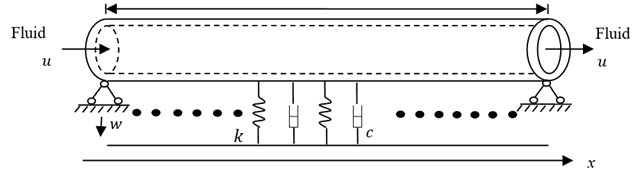a)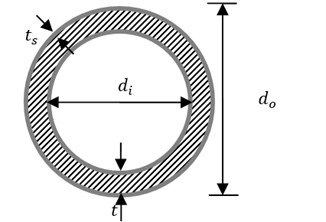b)

Surface effects on the mechanical behaviour of nanomaterials can be examined by considering surface energy and surface residual stresses. The surface energy mainly acts as an increased effect on bending rigidity, the effect with surface residual stress acts as distributed transverse loads and can be calculated by Laplace-Young equations . The additional flexural rigidity of nanotube due to the inner and outer surface layers may be written as :

2
$\mathrm{\hslash }=\frac{1}{8}\pi {E}_{s}{t}_{0}\left({d}_{i}^{3}+{d}_{o}^{3}\right),$

where ${E}_{s}$ and ${t}_{0}$ are Young’s modulus and thickness of both surface layers, ${d}_{i}$ and ${d}_{o}$ are the inner and outer diameters of the nanotube, respectively, as shown in Fig. 1(b). The distributed transverse loads induced by the surface residual stress is $q\left(x\right)={\mathrm{\Pi }}_{0}\left({\partial }^{2}w/\partial {x}^{2}\right)$ and the constant ${\mathrm{\Pi }}_{0}$ is given by:

3
${\mathrm{\Pi }}_{0}=2{\tau }_{0}\left({d}_{i}+{d}_{o}\right),$

where ${\tau }_{0}$ is the residual surface tension.

Considering the influence of two surface layers, the equation of motion of the fluid-conveying nanotube embedded in visco-elastic medium can be written as:

4
$\left(EI+\mathrm{\hslash }\right)\frac{{\partial }^{4}w}{\partial {x}^{4}}+\left({m}_{f}{u}^{2}-{\mathrm{\Pi }}_{0}\right)\frac{{\partial }^{2}w}{\partial {x}^{2}}+2{m}_{f}u\frac{{\partial }^{2}w}{\partial x\partial t}+\left(m+{m}_{f}\right)\frac{{\partial }^{2}w}{\partial {t}^{2}}+kw+c\frac{\partial w}{\partial t}$
$\mathrm{}\mathrm{}\mathrm{}\mathrm{}\mathrm{}\mathrm{}-\left({e}_{0}a{\right)}^{2}\frac{{\partial }^{2}}{\partial {x}^{2}}\left[\left({m}_{f}{u}^{2}-{\mathrm{\Pi }}_{0}\right)\frac{{\partial }^{2}w}{\partial {x}^{2}}+2{m}_{f}u\frac{{\partial }^{2}w}{\partial x\partial t}+\left(m+{m}_{f}\right)\frac{{\partial }^{2}w}{\partial {t}^{2}}+kw+c\frac{\partial w}{\partial t}\right]=0.$

Three typically boundary conditions are considered:

(i) A beam simply supported at both ends, i.e. pinned-pinned condition (P-P):

5
$w\left(0,t\right)=w\left(L,t\right)=0,\mathrm{}\mathrm{}\frac{{\partial }^{2}w\left(0,t\right)}{\partial {x}^{2}}=\frac{{\partial }^{2}w\left(L,t\right)}{\partial {x}^{2}}=0.$

(ii) A beam clamped at both ends, i.e. clamped-clamped condition (C-C):

6
$w\left(0,t\right)=w\left(L,t\right)=0,\mathrm{}\mathrm{}\frac{\partial w\left(0,t\right)}{\partial x}=\frac{\partial w\left(L,t\right)}{\partial x}=0.$

(iii) A beam clamped at one end and simply supported at the other end, i.e. clamped-pinned condition (C-P):

7
$w\left(0,t\right)=w\left(L,t\right)=0,\mathrm{}\mathrm{}\frac{{\partial }^{2}w\left(0,t\right)}{\partial {x}^{2}}=\frac{\partial w\left(L,t\right)}{\partial x}=0.$

For simplicity, introducing dimensionless variables as follows:

8
$\xi =\frac{x}{L},\mathrm{}\mathrm{}\mathrm{}\mathrm{}\mathrm{}\eta =\frac{w}{L},\mathrm{}\mathrm{}\mathrm{}\mathrm{}\mathrm{}U={\left(\frac{{m}_{f}}{EI}\right)}^{1}{2}}Lu,\mathrm{}\mathrm{}\mathrm{}\mathrm{}\mathrm{}\tau ={\left(\frac{EI}{m+{m}_{f}}\right)}^{1}{2}}\frac{t}{{L}^{2}},\mathrm{}\mathrm{}\mathrm{}\mathrm{}\mathrm{}\beta =\frac{{m}_{f}}{m+{m}_{f}},$
$K=\frac{k{L}^{4}}{EI},\mathrm{}\mathrm{}\mathrm{}\mathrm{}\mathrm{}C=\frac{c{L}^{2}}{\sqrt{EI\left({m}_{c}+{m}_{f}\right)}},\mathrm{}\mathrm{}\mathrm{}\mathrm{}\mathrm{}{e}_{n}=\frac{{e}_{0}a}{L},\mathrm{}\mathrm{}\mathrm{}\mathrm{}\mathrm{}\alpha =\frac{\mathrm{\hslash }}{EI},\mathrm{}\mathrm{}\mathrm{}\mathrm{}\mathrm{}\gamma =\frac{{\mathrm{\Pi }}_{0}{L}^{2}}{{\pi }^{2}EI}.$

Eq. (4) can be written in the dimensionless form:

9
$\left(1+\alpha -{e}_{n}^{2}\left({U}^{2}-{\pi }^{2}\gamma \right)\right)\frac{{\partial }^{4}\eta }{\partial {\xi }^{4}}-2\sqrt{\beta }{e}_{n}^{2}U\frac{{\partial }^{4}\eta }{\partial {\xi }^{3}\partial \tau }+\left({U}^{2}-{\pi }^{2}\gamma -K{e}_{n}^{2}\right)\frac{{\partial }^{2}\eta }{\partial {\xi }^{2}}$
$\mathrm{}\mathrm{}\mathrm{}\mathrm{}\mathrm{}\mathrm{}-{e}_{n}^{2}\frac{{\partial }^{4}\eta }{\partial {\xi }^{2}\partial {\tau }^{2}}+2\sqrt{\beta }U\frac{{\partial }^{2}\eta }{\partial \xi \partial \tau }-C{e}_{n}^{2}\frac{{\partial }^{3}\eta }{\partial {\xi }^{2}\partial \tau }+C\frac{\partial \eta }{\partial \tau }+K\eta +\frac{{\partial }^{2}\eta }{\partial {\tau }^{2}}=0.$

The boundary conditions are converted to the following:

P-P condition:

10
$\eta \left(0,t\right)=\eta \left(1,t\right)=0,\mathrm{}\mathrm{}\frac{{\partial }^{2}\eta \left(0,t\right)}{\partial {\xi }^{2}}=\frac{{\partial }^{2}\eta \left(1,t\right)}{\partial {\xi }^{2}}=0.$

C-C condition:

11
$\eta \left(0,t\right)=\eta \left(1,t\right)=0,\mathrm{}\mathrm{}\frac{\partial \eta \left(0,t\right)}{\partial \xi }=\frac{\partial \eta \left(1,t\right)}{\partial \xi }=0.$

C-P condition:

12
$\eta \left(0,t\right)=\eta \left(1,t\right)=0,\mathrm{}\mathrm{}\frac{\partial \eta \left(0,t\right)}{\partial {\xi }^{2}}=\frac{{\partial }^{2}\eta \left(1,t\right)}{\partial \xi }=0.$

## 3. Numerical results

We use the Differential Quadrature Method (DQM) to obtain the solutions of Eqs. (9)-(12). For calculations convenience, the nanotube with circular cross-section area is assumed to be made of anodic alumina with crystallographic of [1 1 1] direction. The parameters may be chosen as follows:$E=$ 70 GPa, ${\rho }_{t}=$ 2700 kg/m3 (mass density of the tube), ${E}_{s}{t}_{0}=$ 5.1882 N/m, ${\tau }_{0}=$ 0.9108 N/m , ${\rho }_{w}=$ 1000 kg/m3 (mass density of the internal water), ${e}_{0}a=$ 1 nm, $c=\mathrm{}$1.02×10-4 Pa·S, ${d}_{i}=$ 20 nm. According to the work by Farshi et al. , for nanostructures made of anodic alumina material, surface effect maybe the most important factor associated with the size-dependent properties.

Fig. 2 shows the variation of the first natural frequency with respect to the dimensionless flow velocity, for different boundary conditions and thicknesses ($t=\left({d}_{o}-{d}_{i}\right)/2$) of the nanotube. It can be seen that the natural frequency decreases as the flow velocity increases and the nanotube buckles when the flow velocity increase to a critical value. The results demonstrate that as the bending stiffness of the nanotube rises from P-P to C-C, the corresponding natural frequencies and the dimensionless critical flow velocities increase. It can be observed that surface effects generally increase the natural frequency and critical flow velocity comparing to the counterpart without surface effects especially for smaller tube thickness. The thickness has a negative influence on the natural frequency, i.e., the smaller the thickness, the larger the natural frequency.

Fig. 2Natural frequency as functions of dimensionless flow velocity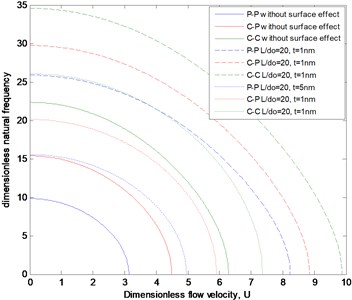Fig. 3Critical flow velocity as functions of tube thickness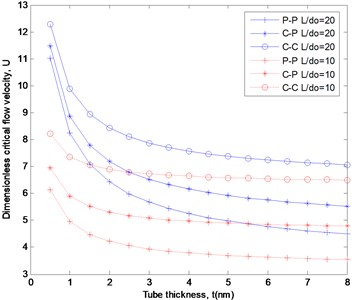Fig. 3 shows the surface effects on the critical flow velocity, for various values of tube thickness and different aspect ratios. It is found that the influence of surface layers on the critical flow velocity is significant, especially for nanotubes with smaller tube thickness or larger aspect ratio. For example, in the case of $L/{d}_{o}=$ 20 and $t=$ 0.5 nm, the critical flow velocity predicted with surface layers is about 3.51 times than that predicted without surface layers, which implies that the surface layers enhance the stability of fluid-conveying nanotubes. The influence of surface layers on critical flow velocity decreases as the tube thickness increases.

Fig. 4Critical flow velocity as functions of dimensionless damping coefficient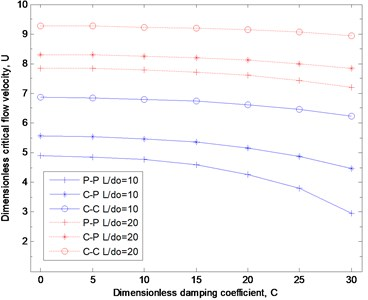Fig. 5Critical flow velocity as functions of dimensionless Winkler parameter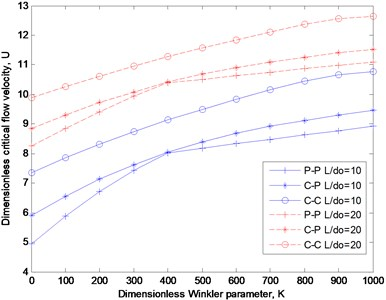The effect of the surrounding visco-elastic medium on the critical flow velocity of nanotube is shown in Fig. 4 and Fig. 5 for different aspect ratios. The dimensionless parameters $C$ and $K$ are used to demonstrate the effects of the visco-elastic behavior of the foundation. It can be concluded from Figs. 4 and 5 that the critical velocity is significantly influenced by the damping and elastic parameter especially for smaller aspect ratio. By increasing the dimensionless damping coefficient $C$ or decreasing the dimensionless elastic parameter $K$, the dimensionless critical flow velocity decreases. This implies that a higher damping property dissipates the vibrational energy quickly and the resonant frequency vanishes at a lower flow velocity, and the elastic parameter enhance the stability of the fluid-conveying nanotube.

## 4. Conclusions

In summary, an analytical model is developed to investigate the surface effects on the vibration and stability analysis of fluid-conveying nanotubes embedded in visco-elastic medium. The results show that the damping and elastic coefficient of medium, thickness of nanotubes and aspect ratios have significant effects on the dynamic behaviors of the nanotubes. This article might be helpful for the design and improvement of nanotubes for fluid-conveying applications embedded in elastic medium in NEMS and MEMS.